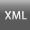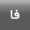Volume 17 -Iranian Journal of Medical Education 2017, 17 - : 50-59 Back to browse issues page
Analyzing the Quality of Objective Structured Clinical Examination in Tehran University of Medical Sciences
Tehran University of Medical Sciences , mjalili@tums.ac.ir
Abstract:   (2357 Views)

Introduction: With the  increasing popularity of performance-based assessments, it is necessary to employ proper indicators to ensure their quality. The present study examines the metrics of Objective Structured Clinical Examination (OSCE).

Methods: In this descriptive, cross-sectional research in 10 pre-internship OSCE stations of Tehran University of Medical Sciences in 2012, the cut-off score was determined by borderline regression method and the failure rate was calculated. Root Mean Square Error (RMSE) was used to estimate error rate threshold.  Internal consistency was calculated using Cronbach’s alpha and then, the Standard Error of Measurement (SEM) was determined. Alpha if item deleted, R2 coefficient, intergrade discrimination, difficulty and discrimination indices were calculated for each station. A total of 266 students participated in this exam.

Results: A total of 266 students participated in this exam. The OSCE total cut-off score was 52.55 (out of 100). Four students (1.5%) failed the exam and the RMSE equaled 0.45. SEM was 4.84 and Cronbach’s alpha was calculated at 0.70 where the alpha if item deleted scores varied from 0.64 to 0.70. The R2 coefficient ranged from 0.16 to 0.85 and the intergrade discrimination ranged between 0.66 and 1.93. The ranges of difficulty and discrimination indices were 0.71-0.89 and 0.12-0.44 respectively.

Conclusion: The internal consistency, SEM and threshold error rate were all acceptable. The alpha if item deleted was in the acceptable range in all stations. In two stations, the R2 value was lower than the desired range. The intergrade discrimination value was appropriate in all stations except one. The stations were not too difficult or highly discriminative which is considered favorable in criterion-referenced exams.

Keywords: Test analysis, psychometrics, Objective Structured Clinical Examination